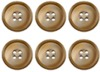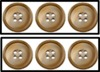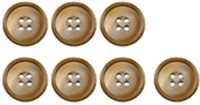# Buttons odd and even

Alignments to Content Standards: 2.OA.C.36 is even.We can write 3+3=6 to show this.7 is odd.We can write 3+3+1=7 to show this.

Count out 20 buttons.

1. Take 8 buttons and count them by twos to determine if there is an even or odd amount. Write an equation showing if it is even or odd.
2. Take 13 buttons and count them by twos to determine if there is an even or odd amount. Write an equation showing if it is even or odd.
3. Take 18 buttons and count them by twos to determine if there is an even or odd amount. Write an equation showing if it is even or odd.

## IM Commentary

The purpose of this task is for students to determine whether a set of objects (up to 20) has an odd or even number of elements. Students will also write an equation to express an even number as a sum of two equal addends and an odd number as a sum of two equal addends and 1. The goal of writing out an equation for each number is that students experience odd numbers as doubles plus one. To assist in this objective teachers will support students in writing the equations for odd numbers as n + n + 1 instead of n + (n+1).

Students should be given 20 buttons to start and will count out the correct number of buttons for each equation.

Some students will easily be able to convert the numbers into equations after counting by two's. Other students may need to physically manipulate the buttons into different groups in order to figure out how to write the correct equation. Either way is acceptable and students should be supported in whichever stage they are at.

## Solution

a. 8 is an even number

4 + 4 = 8

b. 13 is an odd number

6 + 6 + 1 = 13

c. 18 is an even number

9 + 9 = 18